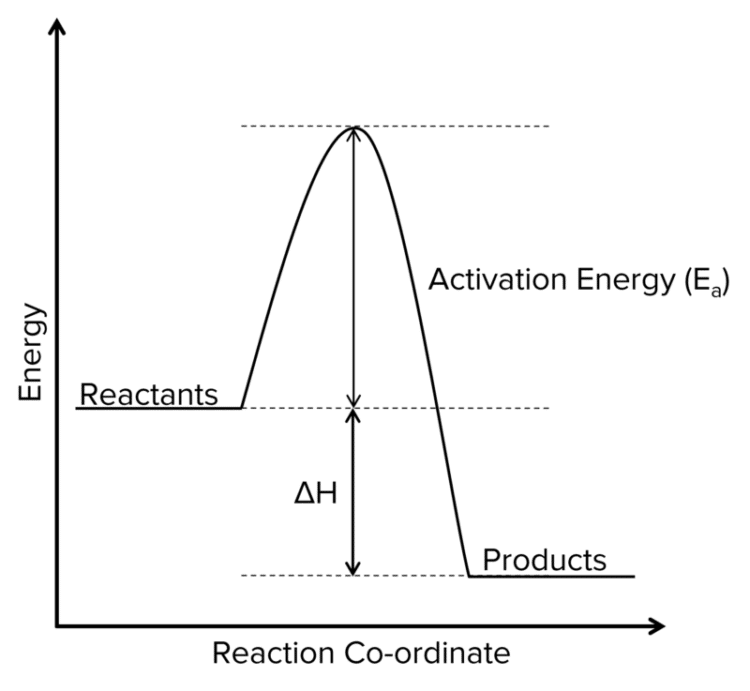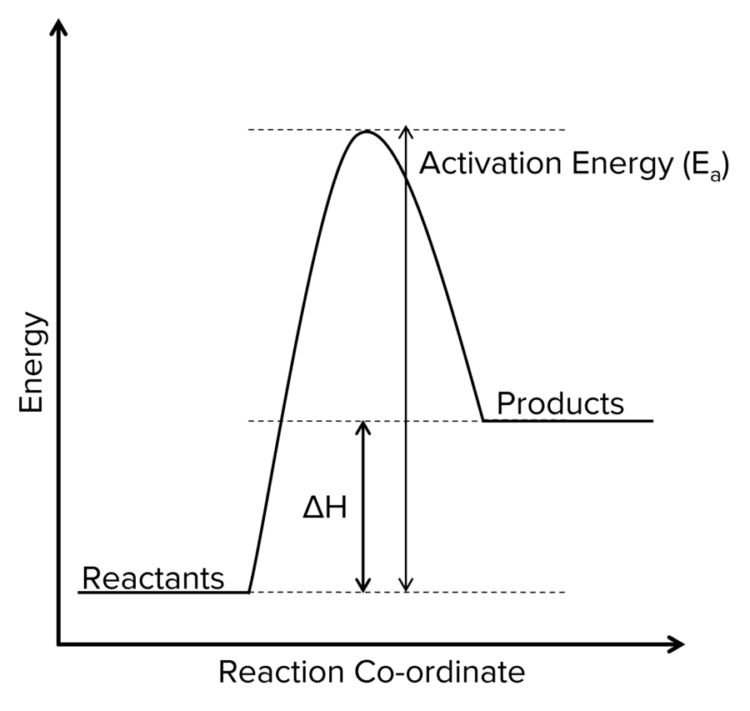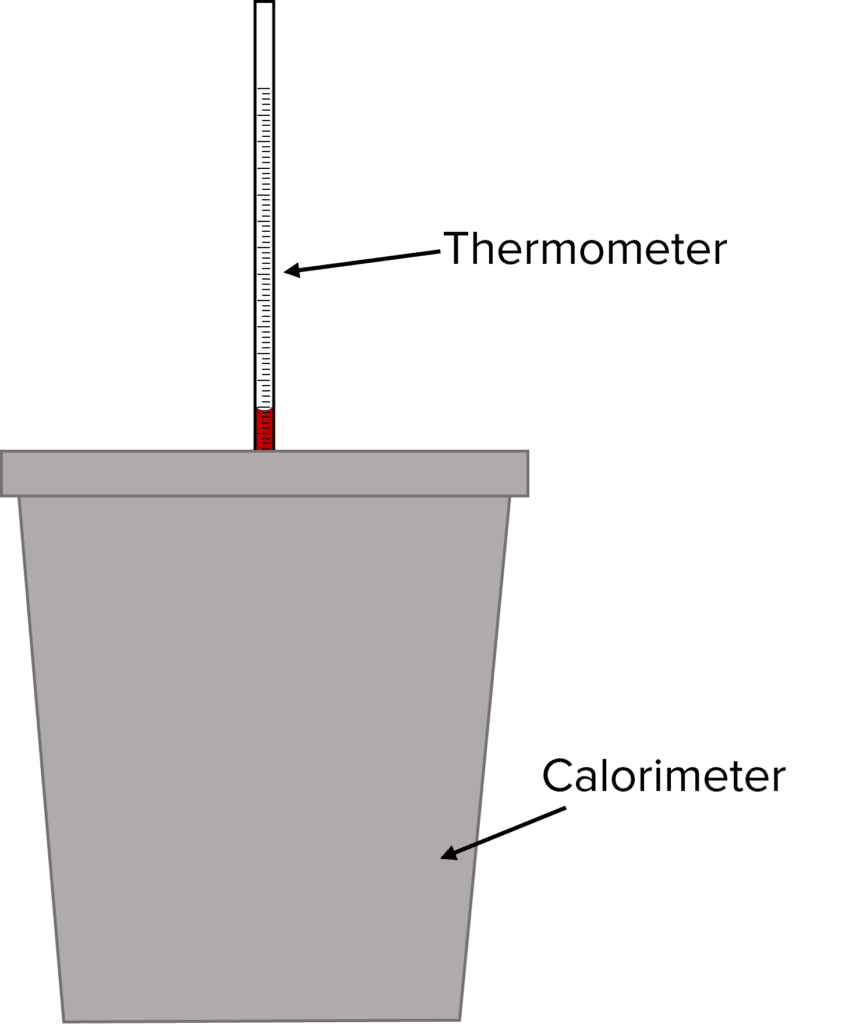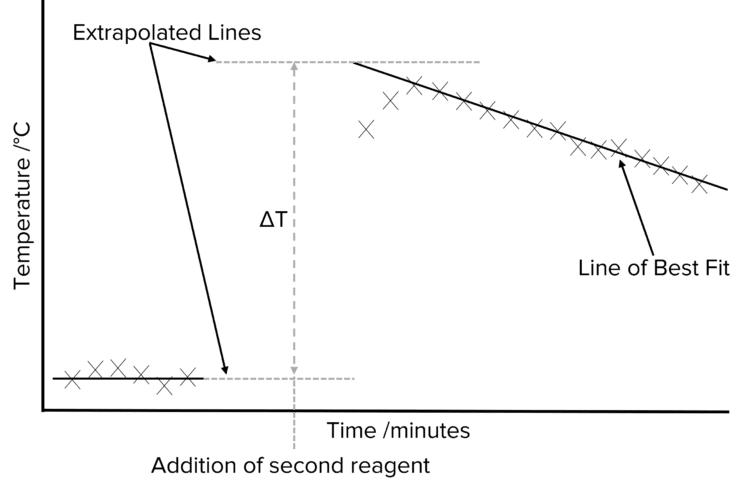# Enthalpy Changes and Calorimetry

A LevelAS LevelAQA

## Enthalpy Changes and Calorimetry

Enthalpy change, $\Delta\text{H}$, is the amount of heat energy that is taken in or given out during a change in a system. Energy is usually transferred between the system and its surroundings. There are many different types of enthalpy changes. The sign and magnitude of these changes will vary from process to process. The study of energy and enthalpy changes is known as thermodynamics.

## Thermodynamics and the System

The study of thermodynamics is a central pillar in chemistry. It allows to understand some of the most important forces that influence chemical processes, including whether or not a given chemical reaction is possible. However, the complexity of energy changes can quickly become overwhelming if the scope of what is being studied is not carefully defined. To do this, scientist consider a idea called the system

The system in thermodynamics is simply the process being studied and the container in which it is taking place. A reaction taking place in a a test tube in a laboratory is an example of a system. It is the energy changes of the system that thermodynamics aims to study.

These energy changes thought of in terms of heat moving into or out of the system from the surroundings. The surroundings in thermodynamics refers to the space in which the system operates. Continuing the example of a test tube reaction, the laboratory in which the reaction is preformed would be the surroundings. Taken together, the system and the surroundings comprise the universe.It is possible to classify reactions according to whether or not they release energy from the system, or take it in. this gives rise to the idea of exothermic and endothermic reactions:

Exothermic: A reaction that releases energy into the surroundings.

Endothermic: A reaction the takes in energy from the surroundings.

The enthalpy changes associated with exothermic reactions are always negative, the temperature changes will always be positive (i.e. exothermic reactions give out heat). The enthalpy changes associated with endothermic reactions are always positive and the temperature changes will always be negative (i.e. endothermic reactions absorb heat).

A LevelAS LevelAQA

## Energy Profiles

Enthalpy changes can be represented using an energy profile diagram. The diagrams show a plot of the energy of a reaction as it progresses through a given reaction co-ordinate. This co-ordinate may be time or movement in physical space, but time is the most common variable.

Reaction profiles will often conform to a general shape. The reactants will be found on the left hand side of the profile, the products on the right. They are typically separated by a peak in the energy curve. This peak represents the activation energy $(\text{E}_a)$ of the reaction.

The position of the reactants and products relative to each other will show whether or not a given reaction is exo or endo-thermic

Exothermic Reaction Profiles• Products have less energy than reactants.
• $\Delta\text{H}$ is negative.
• Temperature increases throughout the reaction.

Endothermic Reaction Profiles•
• Products have more energy than reactants.
• $\Delta\text{H}$ is positive.
• Temperature decreases thoughout the reaction.
A LevelAS LevelAQA

## Standard Enthalpy Change

Enthalpy changes are calculated under standard conditions. Standard conditions are as follows:

• Pressure = $100\text{ kPa}$
• Concentration = $1 \text{mol dm}^{-3}$
• Temperature = $298\text{ K}$ (room temperature)

Standard Enthalpy Change of Formation $(\Delta_{\text{f}}\text{H}^{\varnothing})$The enthalpy change when one mole of a compound is formed from its constituent elements in their standard states under standard conditions.

• The enthalpy of formation for an element is $0$ by definition.

Standard Enthalpy Change of Combustion ($\Delta_{\text{c}}\text{H}^{\varnothing}$)The enthalpy change when one mole of a compound is burned completely in oxygen with all reactants and products in their standard states under standard conditions.

• If the combustion is incomplete, the reaction will be less exothermic.
• Note: By definition, the enthalpy of combustion is $0$.
A LevelAS LevelAQA

## Calorimetric Measurement of an Enthalpy Change

Different experiments can be done to measure enthalpy change. In the calorimetric experiment, substances are mixed in an insulated container, known as a calorimeter, and the temperature of the container is measured over a period of time. The temperature data is then plotted, and extrapolation used to determine the temperature change on reaction of the substances. In the following example, a closed polystyrene cup is used for a calorimeter.Method:

1. Wash and dry the equipment with the solutions that are going to be used.
2. Put a polystyrene cup in a glass beaker for insulation and support.
3. Measure out the volume of $\text{HCl}$ using a pipette and the weight out a sample of the $\text{NaOH}$ using a balance.
4. Put the $\text{HCl}$ solution into the cup.
5. Clamp the thermometer into place and make sure the thermometer is sufficiently immersed in the solution.
6. Measure the initial temperature of the solution and do this every minute for $2-3\text{ minutes}$. If more than one solution is used, do this for the other solution too.
7. Add the $\text{NaOH}$ into the solution.
8. Stir the mixture.
9. Record the temperature each minute for at least $10$ minutes.

AnalysisIf the reaction is slow, then it will be difficult to measure temperature change since cooling and heating would be occurring at the same time so we extrapolate.

We first plot the collected temperature data against time. This will usually give us a set of points at low temperatures – corresponding to the data collected before the addiction of the second reagent) and a set a high temperatures – corresponding to the data collected after the addiction of the second reagent) Two lines of best fit should be drawn, one for the lower temperature data, and one for the higher temperature data. These lines are then be extended (extrapolated) so that they reach the time at which the second reagent was added to the container.

The difference in temperature between the extrapolated lines can then be determined. This gives us the temperature change of reaction, allowing us to calculate the enthalpy change of reaction

Errors in the Calorimetric Method

• There is usually a loss of energy since heat is transferred to the surroundings.
• The reaction could be incomplete.
• We assume that the solutions have the same density and heat capacity as water.
• The specific heat capacity of the calorimeter is not taken into account.

Once the results of the experiment have been collated, we can use the values obtained, such as the temperature change, to calculate the enthalpy change. The sources of error listed above will typically mean that enthalpies calculated from experimental data will be lower than the actual value.

A LevelAS LevelAQA

## Calculating Enthalpy Change

Through carrying out experiments, we can calculate the enthalpy change of a reaction.

\begin{aligned}&\text{Equation }1:\text{Q}=\text{mc}\triangle\text{T}\\&\text{Equation }2:\triangle\text{H}=\dfrac{\text{Q}}{\text{moles}}\end{aligned}

Units

• $\text{Q}$ stands for energy change which is measured in Joules $(\text{J})$
• $\text{m}$ stands for mass of solution which is measured in grams $(\text{g})$
• $\text{c}$ stands for specific heat capacity which is measured in Joules per Kelvin per gram $(\text{J K}^{-1}\text{ g}^{-1})$
• $\triangle\text{T}$ stands for temperature change which is measured in Kelvin $(\text{K})$
• $\triangle\text{H}$ stands for enthalpy change which is measured in kilojoules per mole $(\text{kJ mol}^{-1})$

1. Use equation 1 to work out the energy change in a reaction.
2. Work out the moles used in the reaction
3. Use equation 2 to work out the enthalpy change
4. Depending on if the reaction is endothermic or exothermic, add a sign and units
A LevelAS LevelAQA

## Example: Enthalpy change

Calculate the enthalpy change of a reaction where $\textcolor{#00bfa8}{0.0235\text{ mol}}$ of pure anhydrous copper (II) sulfate is added to $\textcolor{#f21cc2}{25.0\text{ cm}^3}$ of deionised water in a polystyrene cup. An exothermic reaction occurred and the temperature of the water increased by $\textcolor{#a233ff}{150\degree\text{C}}$. The specific heat capacity of water is $\textcolor{#eb6517}{4.18\text{ J K}^{-1}\text{ g}^{-1}}$.

Calculate the enthalpy change, in $\text{kJ mol}^{-1}$, for this reaction.

[3 marks]

$\text{Equation }1:\text{Q}=\text{mc}\triangle\text{T}$

$\text{Q}=\textcolor{#f21cc2}{25}\times4.18\times\textcolor{#a233ff}{15}=\textcolor{#008d65}{1567.5\text{ J}}$

Since we have been given the moles, we can work out enthalpy change immediately.

$\triangle\text{H}=\dfrac{1567.5}{\textcolor{#00bfa8}{0.0235}}=\textcolor{#008d65}{-66.7\text{ kJ mol}^{-1}}$

The answer must be negative since the reaction is exothermic.

Note: The heat capacity of water is always given to you in the question and remains at $4.18\text{ kJ K}^{-1}\text{g}^{-1}$. It is also assumed that all the solutions have the same density as water $\left(1\text{ g cm}^{-3}\right)$, so $50\text{ cm}^3$ will have a mass of $50\text{ g}$.

A LevelAS LevelAQA

## Enthalpy Changes and Calorimetry Example Questions

• Enthalpy change when $1 \text{ mol}$ of compound.
• Is formed from its constituent elements.
• With all substances in their standard states.

$\text{Equation 1: }\text{Q}=\text{mc}\triangle\text{T}$

$\text{Equation 2: Q}=150\times4.18\times8=5016\text{ J}$

$\Delta\text{H}=\dfrac{5016}{0.018}=-279\text{ kJ mol}^{-1}$

$\text{Moles of KCl}=\dfrac{6}{74.6}=0.08042$

$\text{Q}=\triangle\text{H}\times\text{moles}= 17.2\times0.08042=1.383\times\text{kJ}$

$\text{Equation }1:\text{Q}=\text{mc}\triangle\text{T}$

$1.383\times1000=30\times4.18\times\triangle\text{T}$

$\triangle\text{T}=\dfrac{1.383\times1000}{30\times4.18}=11.03\text{ K}$

Enthalpy change is positive so temperature change must be negative.

$\text{T}= 298-11.03=286\text{ K}$

## You May Also Like...### MME Learning Portal

Online exams, practice questions and revision videos for every GCSE level 9-1 topic! No fees, no trial period, just totally free access to the UK’s best GCSE maths revision platform.

£0.00